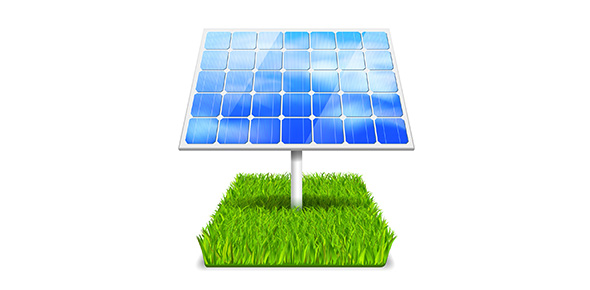38 Questions | Total Attempts: 34SettingsPotential and Kinetic Energy Assessment

• 1.
According to the Law of Conservation of Energy, mechanical energy can be changed to heat energy.
• A.

True

• B.

False

• 2.
The greater the mass a moving object has, the more kinetic energy it has.
• A.

True

• B.

False

• 3.
A rock at the edge of a cliff has kinetic energy because of its position.
• A.

True

• B.

False

• 4.
For work to be done, motion must be in the direction of the applied force.
• A.

True

• B.

False

• 5.
Energy that is stored is kinetic energy.
• A.

True

• B.

False

• 6.
Potential energy depends on position and speed.
• A.

True

• B.

False

• 7.
The units used for work are joules
• A.

True

• B.

False

• 8.
The transfer of energy through motion is called velocity.
• A.

True

• B.

False

• 9.
The ____ energy of an object increases with its height above the ground
• A.

Kinetic

• B.

Mechanical

• C.

Potential

• D.

Heat

• 10.
The kinetic energy of an object increases as its ________ increases
• A.

Specific Heat

• B.

Velocity

• C.

Potential Energy

• D.

Volume

• 11.
A scientist can calculate the amount of work done by using the equation ______.
• A.

W = F x D

• B.

W = F x J

• C.

W = N x m

• D.

W = J x D

• 12.
The amount of work done when a force of one newton acts through a distance of one meter is equal to a _________.
• A.

Kilogram

• B.

Calorie

• C.

Calorie

• D.

Joule

• 13.
If the velocity of an object increases, the _____ of the object will also increase.
• A.

Mass

• B.

Specific Heat

• C.

Kinetic Energy

• D.

Potential Energy

• 14.
Which of the following does not represent work done on a rock in the scientific sense?
• A.

Lifting a rock

• B.

Throwing a rock

• C.

Holding a rock

• D.

Dropping a rock

• 15.
The total amount of kinetic and potential energy in a closed system is called ____.
• A.

Mechanical Energy

• B.

Total Energy

• C.

Summative Energy

• D.

Combined Energy

• 16.
Kinetic energy is directly related to ___/.
• A.

Volume

• B.

Force

• C.

Mass

• D.

Position

• 17.
Potential energy that is depended upon position is called _____
• A.

Chemical potential energy

• B.

Gravitational potential energy

• C.

Positional potential energy

• D.

Conditional potential energy

• 18.
Energy stored in chemical bonds is called _______.
• A.

Gravitational potential energy

• B.

Bonded potential energy

• C.

Chemical potential energy

• D.

Chemically bonded potential energy

• 19.
A train traveling on level tracks at 100 kph.
• A.

Kinetic Energy

• B.

Gravitational Potential Energy

• C.

Chemical Potential Energy

• D.

Both Gravitational and Chemical Potential Energy

• 20.
A cup of milk sitting on a table is an example of ______.
• A.

Kinetic Energy

• B.

Gravitational Potential Energy

• C.

Chemical Potential Energy

• D.

Both Chemical and Gravitational Energy

• 21.
Place the locations on the diagram in order from the greatest to least amount of potential energy.
• A.

W, Z, Y, X

• B.

Y, W, Z, X

• C.

W, Y, Z, X

• D.

W, X, Y, Z

• 22.
Which point on the track has the most kinetic energy?
• A.

W

• B.

X

• C.

Y

• D.

Z

• 23.
Which best describes the energy at point W?
• A.

High PE Low KE

• B.

High PE High KE

• C.

Low PE High KE

• D.

Low PE Low KE

• E.

PE=KE

• 24.
Gasoline is an example of ______.
• A.

Kinetic Energy

• B.

Gravitational Potential Energy

• C.

Chemical Potential Energy

• D.

Both Gravitational and Potential Energy

• 25.
An acorn hanging from a branch of an oak tree waiting to be eaten by a squirrel.
• A.

Kinetic Energy

• B.

Gravitational Potential Energy

• C.

Chemical Potential Energy

• D.

Both Gravitational and Chemical Potential Energy

Related TopicsBack to top